# VLOOKUP Exact Match & Approximate MatchAgnes Lo

## What is VLOOKUP?

Vertical lookup, often known as VLOOKUP, is one of the most popular functions in Excel. It’s specifically designed to navigate through database sources and tables to retrieve data.

The function performs vertical searches down a specific column by looking for a value in the first column of a table and returning a value on the same row in a specific column.

In this article, we'll be exploring VLOOKUP exact match and VLOOKUP approximate match.

### VLOOKUP syntax

Here’s the syntax of VLOOKUP:

``=VLOOKUP(lookup_value, table_array, col_index_num, [range_lookup])``

Now, let’s look into the meaning behind each argument before we go through examples:

#### Lookup_value

The value to lookup in the leftmost column of a table.

#### Table_array

The range of cells that contains the data.

#### Col_index_num

The number of the column in the table, from which the matching value must be returned. The first column is 1, the second column is 2, and so on.

#### [Range_lookup]

It’s optional — not necessary to enter.

### Exact match vs approximate match

Should you wish to specify, there are two options:

1. FALSE to search for an exact match
2. TRUE to find an approximate match.

TRUE is the default value if no specific instruction is given.

Use this free Excel file to practice along with the tutorial

## VLOOKUP example

A customer data analyst has been given the data set below and asked to look up the city of three particular clients by using their client ID, under column G.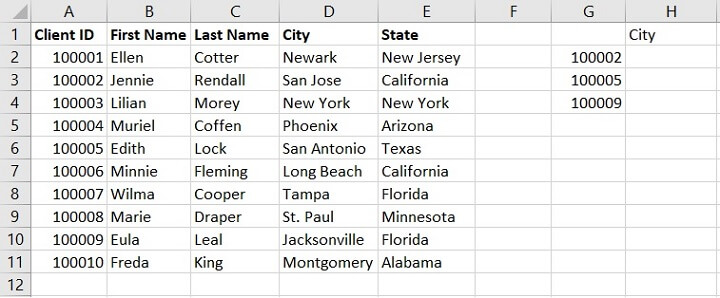To compose the formula in cell H2, let’s break it down in arguments:

#### Lookup_value

100002 is the first client to lookup, so the lookup_value is cell G2.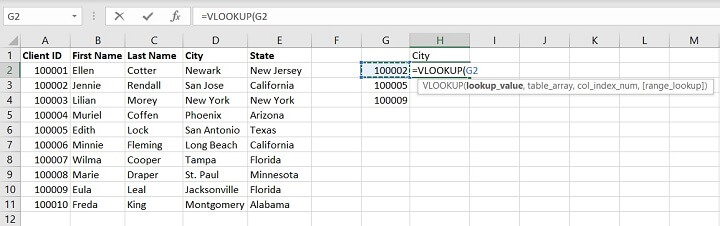#### Table_array

The table from which the value is available is within A1:E11.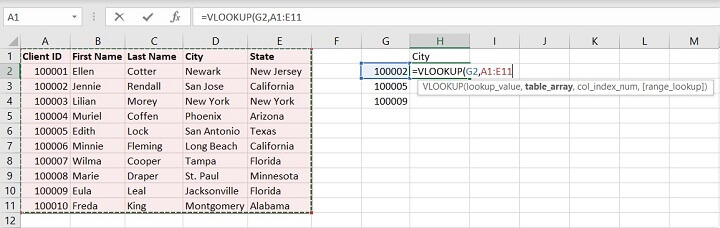#### Col_index_num

There are five columns in total within the table. Information related to the city is under Column D, or the fourth column from the leftmost column. So, we enter 4 as the argument.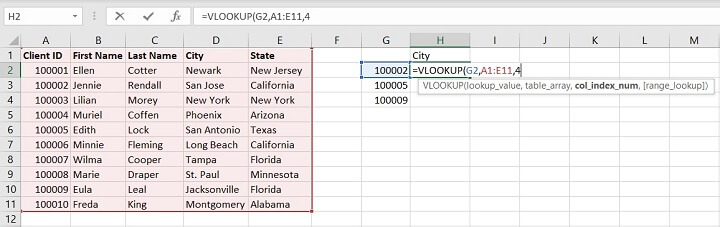#### [Range_lookup]

In the meantime, let’s enter FALSE – exact match.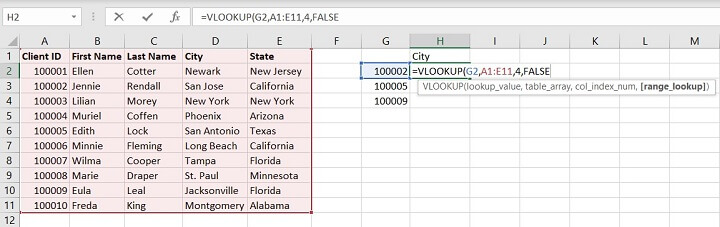The full formula is:

````=VLOOKUP(G2,A1:E11,4,FALSE)`
```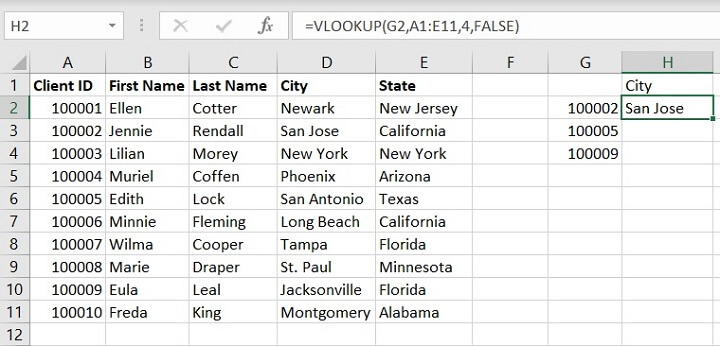## What is exact match in VLOOKUP and how to use it

In most scenarios, exact match is employed when using VLOOKUP, especially when a unique value is the lookup value.

Given that the lookup value is available in the leftmost column of the table provided, an exact match is employed in this VLOOKUP example.

This time VLOOKUP is to lookup value 20606 or E2 in the leftmost column of the table array given — A:C.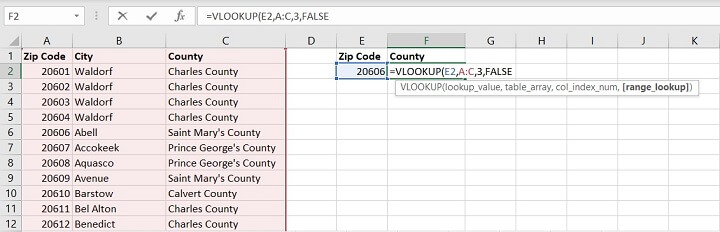Since the corresponding value to lookup is in the third column of the table, we enter value 3 to tell Excel to return the value in the same row from the third column of the table array.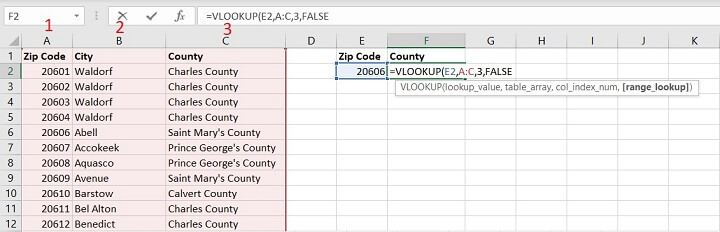By entering FALSE in the fourth argument, we inform Excel to return an exact match.

Excel returns Saint Mary’s County, which is a correct corresponding value to zip code 20606.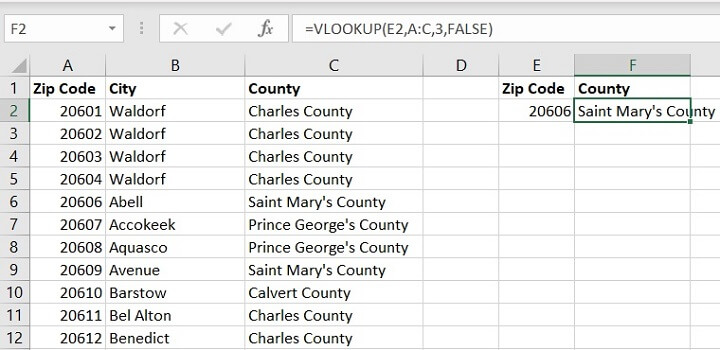If the lookup value is not available in the leftmost column of a table, Excel will return #N/A as the value is not found.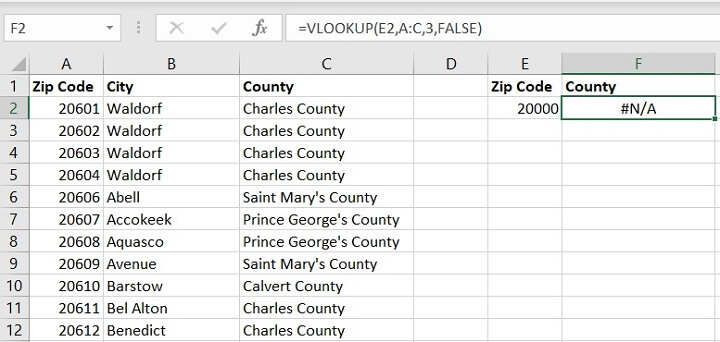## What is approximate match in VLOOKUP and how to use it

Approximate match is the default range_lookup in the formula. When no instruction is given, Excel assumes the lookup refers to an approximate match.

In most cases, it’s less frequently used compared to exact match, though it is useful when the lookup value is not available within the table array.

For instance, the annual income of \$39,000 is not available in the leftmost column of the table.  In this situation, TRUE is useful in requesting Excel to return an approximate match. As \$39,000 is not available, it will return a value smaller than \$39,000 (\$30,000 in this example) — 8% of salary tax.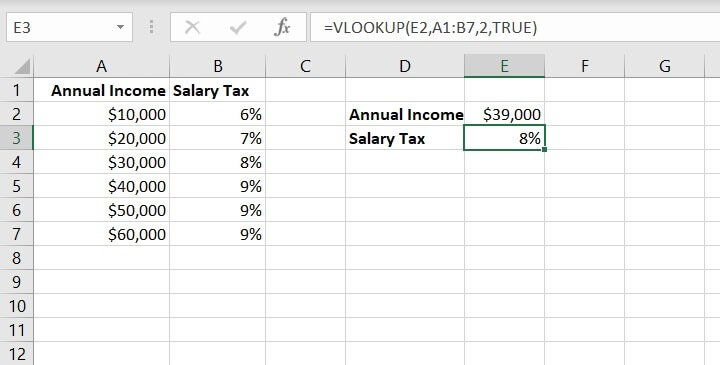If we use FALSE (exact match in this situation), since there’s no value of \$39,000 available in the leftmost column, Excel will return #N/A.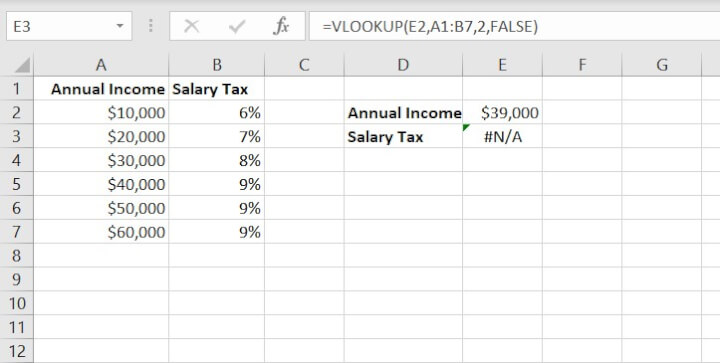## VLOOKUP tips

### 1.  VLOOKUP looks to the right

VLOOKUP always looks to the right. It only looks up a value in the leftmost column of a table, and returns a corresponding value to the right in the same row.

For example, VLOOKUP is able to lookup the client ID and return the corresponding first name, last name, city and state — all the information to the right of the lookup value.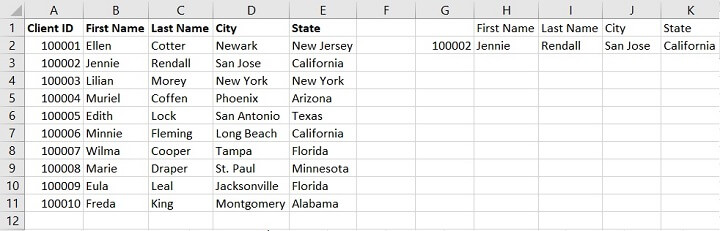### 2.  First match

If there are duplicates in the leftmost column of the table, VLOOKUP always returns the first match only.

In the example below, both Lilian and Muriel have the Client ID 100003. VLOOKUP returns Lilian instead of Muriel, as Lilian is the first available value.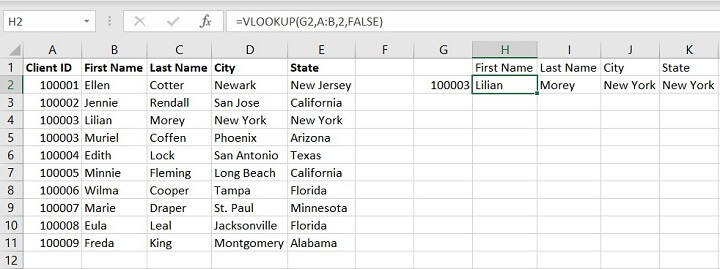If we swap row 4 & 5, with Muriel on top of Lilian, VLOOKUP returns Muriel as the return value.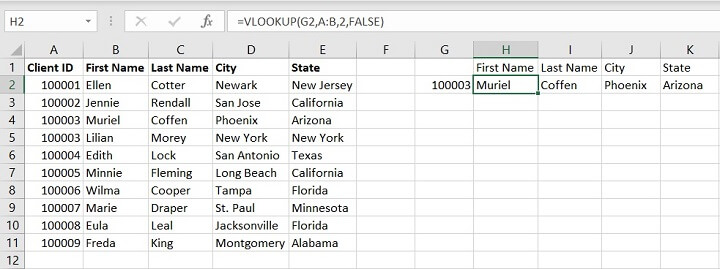## Summary

VLOOKUP is one of the most used functions in Excel. It searches through a given data set based on a lookup value in the leftmost column and returns a corresponding value in the same row.

In most situations, where the lookup value is a unique known identifier, exact match is used to return the exact corresponding information in the same row. In some occasions, where the lookup value is not unknown, approximate match is used to find the closest match, given the criteria.

Though it may look difficult to set up in the beginning, VLOOKUP is very useful when it comes to searching for information when handling multiple data sets. It’s important to know that VLOOKUP only works by looking to the right for matching information and returns the first match only.

To learn more about VLOOKUP and other essential Excel functions, enroll in our Microsoft Excel - Basic and Advanced course today. Try it for free with a 7 day free trial to all courses on GoSkills.

Ready to become a certified Excel ninja?